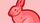cancel
Showing results for
Did you mean:

## SHA512 - Signature Key to Byte Array Java

Authorize.NET provides C# code to convert the Signature Key to a Byte Array and calculate the HMAC-SHA512 value.  Their example code is using lambda coding in C#.  Can someone provide a Java equivalent example of this?  I have  non-lambda Java version working, but I would like to know how to do a Java lambda version.

I came up with the following, but it won't work because the .toArray() method returns an int array, not a byte array:

byte[] k = java.util.stream.IntStream.range(0, binSigKey.length)

.filter(i -> i % 2 == 0)

.map(i -> Byte.parseByte(signatureKey.substring(i, i + 2), 16))

.toArray();

Original C# version provided by Authorize.NET as an example:

byte[] k = Enumerable.Range(0, key.Length)
.Where(x => x % 2 == 0)
.Select(x => Convert.ToByte(key.Substring(x, 2), 16))
.ToArray();

Thanks.Member
0 REPLIES 0